# Sphere

Jump to: navigation, search

## Definition

### As a subset of Euclidean space

The unit$n$-sphere$S^n$ is defined as the subset of Euclidean space$\R^{n+1}$ comprising those points whose distance from the origin is$1$.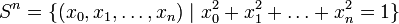$S^n = \{ (x_0,x_1,\dots,x_n) \mid x_0^2 + x_1^2 + \dots + x_n^2 = 1 \}$

### Inductive definition

Inductively,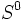$S^0$ is defined as a discrete two-point space, and for any natural number$n$,$S^n$ is defined as the suspension of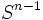$S^{n-1}$.

This definition is illuminative because many of the results about spheres, particularly those involving algebraic topology and the computation of homology and cohomology, are easily derived from corresponding results about suspensions.

## Particular cases$n$ sphere$S^n$
0$S^0$ -- discrete two-point space
1 circle
2 2-sphere
3 3-sphere

## Equivalent spaces

Space How strongly is it equivalent to the circle?
boundary of the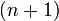$(n+1)$-hypercube homeomorphic; not diffeomorphic because of sharp edges
boundary of the$(n+1)$-simplex homeomorphic; not diffeomorphic because of sharp edges
ellipsoid in$\R^{n+1}$ equivalent via affine transformation
one-point compactification of$\R^n$ homeomorphic via stereographic projection
for$n \ge 2$: universal cover of real projective space$\R\mathbb{P}^n$, which is the space of lines in$\R^{n+1}$ homeomorphic, diffeomorphic, also isometric if we choose the natural metric.

## Algebraic topology

### Homology groups

Further information: homology of spheres

With coefficients in$\mathbb{Z}$, the$n$-sphere$S^n$ has$H_0(S^n) \cong H_n(S^n) \cong \mathbb{Z}$ and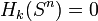$H_k(S^n) = 0$ for$k \notin \{ 0, n \}$. In particular, the$n$-sphere is$(n - 1)$-connected.

Interpretations in terms of various homology theories:

Fill this in later

With coefficients in any$R$-module$M$ for a ring$R$, the$n$-sphere$S^n$ has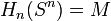$H_n(S^n) = M$ and$H_k(S^n) = 0$ for all$k \ne n$.

### Cohomology groups and cohomology ring

Further information: cohomology of spheres

With coefficients in$\mathbb{Z}$, the$n$-sphere$S^n$ has$H^0(S^n) \cong H^n(S^n) \cong \mathbb{Z}$ and$H^k(S^n) = 0$ for$k \notin \{ 0,n\}$. In particular, the$n$-sphere is$(n - 1)$-connected.

With coefficients in any$R$-module$M$ for a ring$R$, the$n$-sphere$S^n$ has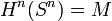$H^n(S^n) = M$ and$H^k(S^n) = 0$ for all$k \ne n$.

The cohomology ring is isomorphic to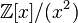$\mathbb{Z}[x]/(x^2)$, where$x$ is a generator of the$n^{th}$ cohomology.

### Homotopy groups

Further information: homotopy of spheres, n-sphere is (n-1)-connected

For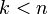$k < n$, the homotopy group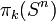$\pi_k(S^n)$ is the trivial group.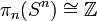$\pi_n(S^n) \cong \mathbb{Z}$, with the identity map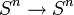$S^n \to S^n$ being a generator.

The cases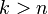$k > n$ are discussed below:

Case What can we say?$n = 1$$\pi_k(S^n)$ is trivial for all$k > 1$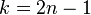$k = 2n - 1$$\pi_k(S^n) \cong \mathbb{Z}$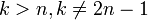$k > n, k \ne 2n - 1$$\pi_k(S^n)$ is a finite abelian group